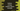# Python numpy.random.choice method example## Python numpy.random.choice example to generate a random sample from a given 1-D array:

numpy.random.choice method is used to generate a random sample from a 1-D array. It gets the random sample from a 1-D array and returns that random sample.

In this post, we will learn how to use numpy.random.choice() with examples.

## Definition of numpy.random.choice():

numpy.random.choice() method is defined as like below:

``choice(arr, size, replace, p)``

Here,

• arr is 1-D array-like or int. If we pass an integer, it takes the np.arrange value for that integer.
• size is optional. It is used to define the output shape. By default it is None and it returns a single value. It can be a int or tuple of ints.
• replace is optional boolean value. It defines whether replacement is supported or not. By default, it is True, i.e. a value of arr can be selected multiple times.
• p is 1-D array-like optional parameter. It is the probabilities associated with each entries.

### Example of random.choice():

Let’s pass one integer value as the first param:

``````from numpy import random as r

print(r.choice(3, 4))``````

It will take 3 as numpy.arrange(3) and it will print output as like below:

``[2 0 1 0]``

### Example with replacement False:

We can create a random sample without replacement if we add replace = False parameter.

``````from numpy import random as r

print(r.choice(3, 3, replace=False))``````

It will print one output as like below:

``[0 2 1]``

#### Error while replace=False:

It will throw ValueError is the size is greater than the given sample size.

``````from numpy import random as r

print(r.choice(3, 4, replace=False))``````

It will throw ValueError:

``ValueError: Cannot take a larger sample than population when 'replace=False'``

### Using probability:

We can pass the p parameter to add probabilities to the entries:

``````from numpy import random as r

print(r.choice(4, 2,  p=[0.1, 0.2, 0.5, 0.2]))``````

It will print something as like below:

``[2, 1]``